# NCERT Solutions for Class 10 Science Chapter 3 - Chemical Reactions and Equations

##### Question 1:

Why do we apply paint on iron articles?

The iron articles can be protected from rusting by applying paint on them so that the iron surface does not come in contact with air and moisture which cause rusting.

##### Question 2:

Oil and fat containing food items are flushed with nitrogen. Why?

This is done to prevent the oxidation of food by oxygen or air. Nitrogen, being inert, forms a protective layer over the food items and prevent their contact with air or oxygen.

##### Question 3:

Explain the following terms with one example each:

1. Corrosion
2. Rancidity
1. Corrosion: The slow eating up of metals by the action of air and moisture on their surfaces is called corrosion.
2. Rancidity: When fats and oils or food containing fats and oils are oxidised with air or oxygen, their smell and taste change. This process is called rancidity.
##### Question 4:

Which of the following gases can be used for storage of fresh sample of oil for a long time?

1. Carbon dioxide or helium
2. Carbon dioxide or oxygen
3. Nitrogen or oxygen
4. Helium or nitrogen

Helium or nitrogen

##### Question 5:

Grapes hanging on the plant do not ferment but after being plucked from the plant can be fermented. Under what conditions do these grapes ferment? Is it a chemical or a physical change?

Grapes attached to plants are living and hence their self defense system prevents the growth of the microbes causing fermentation. But in the plucked grapes microbes grow and fermentation takes place. It is a chemical change.

##### Question 6:

A silver article generally turns black when kept in the open for a few days. The article when rubbed with toothpaste again starts shining.

1. Why do silver articles turn black when kept in the open for a few days? Name the phenomenon involved.
2. Name the black substance formed and give its chemical formula.
1. Silver articles turn black because silver reacts with hydrogen sulphide present in air and form a black substance. This substance deposits on the surface of the silver article. This phenomenon is called corrosion.
2. The black substance is silver sulphide and its formula is Ag2S.
##### Question 7:

A solution of substance ‘X’ is used for whitewashing.

1. Name the substance ‘X’ and write its formula.
2. Write the reaction of the substance ‘X’ named in (i) above with water.
1. X is calcium oxide (quick lime) and its formula is CaO.
2. CaO(s) + H2O(l) → Ca(OH)2(aq)
##### Question 8:

Why is the amount of gas collected in one of the test tubes in Activity 7 double of the amount collected in the other? Name this gas.

When electric current is passed through water containing acid, the following reaction takes place: 2H2O(l) → 2H2(g) + O2(g)

As depicted in the equation, hydrogen and oxygen produced are in the ratio 2 :1 by volume. Hence the amount of gas collected in one test tube is double of the amount of gas collected in the other tube. This gas is hydrogen.

##### Question 9:

Why does the colour of copper sulphate solution change, when an iron nail is dipped in it?

When an iron nail is dipped in copper sulphate solution, displacement reaction takes place in which iron displaces copper from copper sulphate solution. Consequently, the concentration of copper sulphate solution decreases and blue colour of the solution gradually fades away.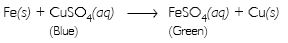##### Question 10:

Give an example of a double displacement reaction other than the one given in Activity 10.

NaCl (aq) + AgNO3(aq) → AgCl(s) + NaNO3(aq)

##### Question 11:

Identify the substances that are oxidised and the substances that are reduced in the following reactions:

1. 4Na(s) + O2(g) → 2Na2O(s)
2. CuO(s) + H2(g) → Cu(s) + H2O(l)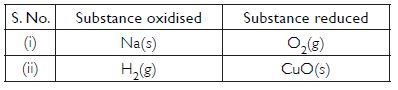##### Question 12:

Which of the statements about the reaction below are incorrect?

2PbO(s) + C(s) → 2Pb(s) + CO2(g)

2. Carbon dioxide is getting oxidised.
3. Carbon is getting oxidised.
4. Lead oxide is getting reduced.
1. (i) and (ii)
2. (i) and (iii)
3. (i), (ii) and (iii)
4. all

(a) (i) and (ii)

##### Question 13:

Fe2O3 + 2Al → Al2O3 + 2Fe

The above reaction is an example of a

1. combination reaction
2. double displacement reaction
3. decomposition reaction
4. displacement reaction

Displacement reaction

##### Question 14:

What does one mean by exothermic and endothermic reactions? Give examples.

Exothermic reactions: Chemical reactions in which heat energy is given out are called exothermic reactions. For example,
C(s) + O2(g) → CO2(g) + Heat energy
NaOH(aq) + HCl(aq) → NaCl(aq) + H2O(l) + Heat energy
Endothermic reactions: Chemical reactions in which heat energy is absorbed are called endothermic reactions. For example,
N2(g) + O2(g) + Heat energy → 2NO(g)
C(s) + H2O(g) + Heat energy → CO(g) + H2(g)

##### Question 15:

Why is respiration considered an exothermic reaction? Explain.

During respiration, oxidation of glucose occurs that produces heat energy. As heat energy is released,respiration is an exothermic reaction.

##### Question 16:

Why are decomposition reactions called opposite of combination reactions? Write equations for these reactions.

During a decomposition reaction, a single substance breaks down into two or more substances. It is just the reverse of a combination reaction in which two or more substances combine to form a single substance.

Examples for decomposition reactions are:

2Pb(NO3)2(s) → 2PbO(s) + 4NO2(g) + O2(g)

2Pb(NO3)2(s) → 2PbO(s) + 4NO2(g) + O2(g)

##### Question 17:

Write one equation each for decomposition reactions, in which energy is supplied in the form of heat, light or electricity.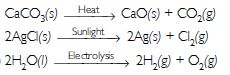##### Question 18:

What is the difference between displacement and double displacement reactions? Write equations for both types of reactions.

Displacement reaction: In a displacement reaction, a more reactive element displaces a less reactive element from a solution of its compound. Examples:

Fe(s) + CuSO4(aq) → FeSO4(aq) + Cu(s)
Zn(s) + CuSO4(aq) → ZnSO4(aq) + Cu(s)

Double displacement reaction: The reaction in which there is an exchange of ions between two reactants is called a double displacement reaction. Examples:

AgNO3(aq) + NaCl(aq) → AgCl(s)↓ + NaNO3(aq) BaCl2(aq) + Na2SO4(aq) → BaSO4(s)↓ + 2NaCl(aq)

##### Question 19:

In the refining of silver, the recovery of silver from silver nitrate solution involves displacement by copper metal. Write down the reaction involved.

Cu(s) + 2AgNO3(aq) → Cu(NO3)2(aq) + 2Ag(↓)

##### Question 20:

What do you mean by precipitation reaction? Explain by giving examples.

A reaction in which an insoluble product or precipitate is formed is called a precipitation reaction.
Examples:
AgNO3(aq) + NaCl(aq) → AgCl(↓) + NaNO3(aq)
BaCl2(aq) + Na2SO4(aq) → BaSO4(↓) + 2NaCl (aq)

##### Question 21:

Explain the following in terms of gain or loss of oxygen with two examples each:

(a) Oxidation (b) Reduction

(a) Oxidation: A chemical reaction in which a substance gains oxygen or loses hydrogen is called oxidation.
Examples:
2Cu + O2 → 2CuO, Cu is oxidised to CuO
2Mg + O2 → 2MgO, Mg is oxidised to MgO
(b) Reduction: A chemical reaction in which a substance loses oxygen or gains hydrogen is called reduction.
ZnO + C → Zn + CO, ZnO is reduced to Zn.
CuO + H2 → Cu + H2O, CuO is reduced to Cu

##### Question 22:

A shiny brown coloured element ‘X’ on heating in air becomes black in colour. Name the element ‘X’ and the black coloured compound formed.

The element X is copper. The black coloured compound formed is copper (II) oxide (CuO).

##### Question 23:

The following reaction is an example of a
4NH3(g) + 5O2(g) → 4NO(g) + 6H2O(g)

1. displacement reaction
2. combination reaction
3. redox reaction
4. neutralisation reaction
1. (ii) and (iii)
2. (iii) and (iv)
3. (i) and (iii)
4. (i) and (iv)

(i) and (iii)

##### Question 24:

Which of the following statements about the given reaction are correct?

3Fe(s) + 4H2O(g) → Fe3O4(s) + 4H2(g)

1. Iron metal is getting oxidised
2. Water is getting reduced
3. Water is acting as reducing agent
4. Water is acting as oxidising agent
1. (ii) and (iv)
2. (i), (ii) and (iv)
3. (iii) and (iv)
4. (i), (ii) and (iii)

(i), (ii) and (iv)

##### Question 25:

Which of the following are exothermic processes?

1. Reaction of water with quick lime
2. Dilution of an acid
3. Evaporation of water
4. Sublimation of camphor (crystals)
1. (i) and (ii)
2. (iii) and (iv)
3. (ii) and (iii)
4. (i) and (iv)

(i) and (ii)

##### Question 26:

Three beakers labelled as A, B and C, each containing 25 ml of water were taken. A small amount of NaOH, anhydrous CuSO4 and NaCl were added to the beakers A, B and C respectively. It was observed that there was an increase in the temperature of the solutions contained in beakers A and B, whereas in case of beaker C, the temperature of the solution falls, which one of the following statement(s) is(are) correct?

1. In beakers A and B, exothermic process has occurred
2. In beakers A and B, endothermic process has occurred
3. In beaker C exothermic process has occurred
4. In beaker C endothermic process has occurred
1. (ii) only
2. (ii) and (iii)
3. (i) and (iv)
4. (i) only

(i) and (iv)

##### Question 27:

Which among the following is (are) double displacement reaction(s)?

1. Pb + CuCl2 → PbCl2 + Cu (ii) Na2SO4 + BaCl2 → BaSO4+ 2NaCl
2. C + O2 → CO2 (iv) CH4 + 2O2 → CO2 + 2H2O
1. (i) and (ii)
2. (ii) only
3. (i) and (iv)
4. (iii) and (iv)

(ii) only

##### Question 28:

Solid calcium oxide reacts vigorously with water to form calcium hydroxide accompanied by liberation of heat. This process is called slaking of lime. Calcium hydroxide dissolves in water to form its solution called lime water. Which among the following is (are) true about slaking of lime and the solution formed?

1. It is an endothermic reaction
2. It is an exothermic reaction
3. The pH of the resulting solution will be more than seven
4. The pH of the resulting solution will be less than seven
1. (iii) and (iv)
2. (i) and (iv)
3. (i) and (ii)
4. (ii) and (iii)

(ii) and (iii)

##### Question 29:

Barium chloride on reacting with ammonium sulphate forms barium sulphate and ammonium chloride. Which of the following correctly represents the type of the reaction involved?

1. Displacement reaction
2. Precipitation reaction
3. Combination reaction
4. Double displacement reaction
1. (iv) only
2. (iv) only
3. (ii) only
4. (ii) and (iv)

(ii) and (iv)

##### Question 30:

Electrolysis of water is a decomposition reaction. The molar ratio of hydrogen and oxygen gases liberated during electrolysis of water is:

1. 1 : 2
2. 2 : 1
3. 1 : 1
4. 4 : 1

2 : 1

##### Question 31:

Which of the following is (are) an endothermic process (es)?

1. Dilution of sulphuric acid
2. Sublimation of dry ice
3. Condensation of water vapour
4. Evaporation of water
1. (iii) only
2. (i) and (iii)
3. only
4. (ii) and (iv)

(ii) and (iv)

##### Question 32:

In the double displacement reaction between aqueous potassium iodide and aqueous lead nitrate, a yellow precipitate of lead iodide is formed. While performing the activity if lead nitrate is not available, which of the following can be used in place of lead nitrate?

1. Ammonium nitrate
3. Potassium sulphate

##### Question 33:

The following reaction is used for the preparation of oxygen gas in the laboratory:
2KClO3(s) → 2KCl(s)+ 3O2(g)
Which of the following statement(s) is (are) correct about the reaction?

1. It is a decomposition reaction and endothermic in nature
2. It is a photochemical decomposition reaction and exothermic in nature
3. It is a decomposition reaction and accompanied by the release of heat
4. It is a combination reaction

It is a decomposition reaction and endothermic in nature

##### Question 34:

Which one of the following processes involves chemical reactions?

1. Keeping petrol in a china dish in the open
2. Storing of oxygen gas under pressure in a gas cylinder
3. Liquefaction of air
4. Heating copper wire in presence of air at high temperature

Heating copper wire in presence of air at high temperature

##### Question 35:

Which of the following are combination reactions?

1. 2KCIO4 Heat 2KCI + 3O2
2. MgO + H2O → Mg(OH)2
3. 4AI + 3O2 → 2Al2O3
4. Zn + FeSO4 → ZnSO4 + Fe
1. (ii) and (iv)
2. (i) and (iii)
3. (iii) and (iv)
4. (ii) and (iii)

(ii) and (iii)

##### Question 36:

Which among the following changes are exothermic or endothermic in nature?

1. Dissolution of ammonium chloride in water
2. Dissolution of sodium hydroxide in water
3. Dilution of sulphuric acid
4. Decomposition of ferrous sulphate
1. Endothermic reaction
2. Exothermic reaction
3. Exothermic reaction
4. Endothermic reaction
##### Question 36:

Identify the reducing agent in the following reactions:

1. 2H2 + O2 → 2H2O
2. Fe2O3 + 3CO → 2Fe + 3CO2
3. H2O + F2 → HF + HOF
4. 4NH3 + 5O2 → 4NO + 6H2O

(a) H2 (b) CO (c) H2O (d) NH3

##### Question 37:

Identify the oxidising agent (oxidant) in the following reactions:

1. CuO + H2 Cu + H2O
2. 3Fe + 4H2O → Fe3O4 + 4H2
3. V2O5 + 5Ca → 2V + 5CaO
4. CuSO4 + Zn → Cu + ZnSO4
5. 2Mg + O2 → 2MgO
6. Pb3O4 + 8HCl → 3PbCl2 + Cl2 + 4H2O
1. CuO
2. H2O
3. V2O5
4. CuSO4
5. O2
6. Pb3O4
##### Question 38:

A solution of potassium chloride when mixed with silver nitrate solution, an insoluble white substance is formed. Write the chemical reaction involved and also mention the type of the chemical reaction.

KCl(aq) + AgNO3(aq) → KNO3(aq) + AgCl(s)

It is double displacement reaction.

##### Question 39:

Ferrous sulphate decomposes with the evolution of a gas having a characteristic odour of burning sulphur. Write the chemical reaction involved and identify the type of reaction.

2FeSO4(s) → Fe2O3(s) + SO2(g) + SO3(g)

It is a thermal decomposition reaction.

##### Question 40:

A substance X, which is an oxide of a group 2 element, is used intensively in the cement industry. This element is present in bones also. On treatment with water it forms a solution which turns red litmus blue. Identify X and also write the chemical reactions involved.

Element X is calcium (Ca). On treatment with water, calcium forms calcium hydroxide, which is basic in nature and turns red litmus blue.
CaO + H2O → Ca(OH)2

##### Question 41:

Write the balanced equation for the following chemical reactions:

1. Hydrogen + Chlorine → Hydrogen chloride
2. Barium chloride + Ammonium sulphate → Barium sulphate + Ammonium chloride
3. Sodium + Water → Sodium hydroxide + Hydrogen
1. H2 + Cl2 → 2HCl
2. BaCl2 + (NH4)2SO4 → BaSO4 + 2NH4Cl
3. 2Na + 2H2O → 2NaOH + H2
##### Question 42:

Write a balanced chemical equation with state symbols for the following reactions:

1. Solutions of barium chloride and sodium sulphate in water react to give insoluble barium sulphate and the solution of sodium chloride.
2. Sodium hydroxide solution (in water) reacts with hydrochloric acid solution (in water) to produce sodium chloride and water.
1. BaCl2(aq) + Na2SO4(aq) → BaSO4(s) + 2NaCl(aq)
2. NaOH(aq) + HCl(aq) → NaCl(aq) + H2O(l)
##### Question 43:

What is a balanced chemical equation? Why should chemical equations be balanced?

Balanced chemical equation is an equation in which number of atoms of each element is equal on both sides of the equation.

The chemical equation should be balanced because, according to the law of conservation of mass, the total mass of the reactants must be equal to the total mass of the products.

##### Question 44:

Translate the following statements into chemical equations and then balance them:

1. Hydrogen gas combines with nitrogen to form ammonia.
2. Hydrogen sulphide gas burns in air to give water and sulphur dioxide.
3. Barium chloride reacts with aluminium sulphate to give aluminium chloride and a precipitate of barium sulphate.
4. Potassium metal reacts with water to give potassium hydroxide and hydrogen gas.
1. 3H2(g) + N2(g) → 2NH3(g)
2. 2H2S(g) + 3O2(g) → 2H2O(l) + 2SO2(g)
3. 3BaCl2(aq) + Al2(SO4)3(s) → 2AlCl3(aq) + 3BaSO4(s)
4. 2K(s) + 2H2O(l) → 2KOH(aq) + H2(g)
##### Question 45:

Balance the following chemical equations: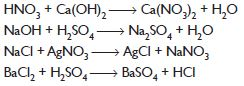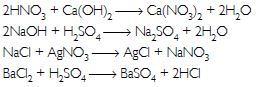##### Question 46:

Write the balanced chemical equations for the following reactions:

1. Calcium hydroxide + Carbon dioxide → Calcium carbonate + Water
2. Zinc + Silver nitrate → Zinc nitrate + Silver
3. Aluminium + Copper chloride → Aluminium chloride + Copper
4. Barium chloride + Potassium sulphate → Barium sulphate + Potassium chloride
1. Ca(OH)2 + CO2 → CaCO3 + H2O
2. Zn + 2AgNO3 → Zn(NO3)2 + 2Ag
3. 2Al + 3CuCl2 → 2AlCl3 + 3Cu
4. BaCl2 + K2SO4 → BaSO4 + 2KCl
##### Question 47:

Write the balanced chemical equation for the following reactions and identify the type of reaction in each case.

1. Potassium bromide (aq) + Barium iodide (aq) → Potassium iodide (aq) + Barium bromide (s)
2. Zinc carbonate (s) → Zinc oxide (s) + Carbon dioxide (g)
3. Hydrogen (g) + Chlorine (g) → Hydrogen chloride (g)
4. Magnesium (s) + Hydrochloric acid (aq) → Magnesium chloride (aq) + Hydrogen (g)
1. 2KBr(aq) + BaI2(aq) → 2KI(aq) + BaBr2(aq) — Double displacement reaction
2. ZnCO3(s) → ZnO(s) + CO2(g) — Decomposition reaction
3. H2(g) + Cl2(g) → 2HCl(g) — Combination reaction
4. Mg(s) + 2HCl(aq) → MgCl2(aq) + H2(g) — Displacement reaction
##### Question 48:

In which of the following chemical equations, the abbreviations represent the correct states of the reactants and products involved at reaction temperature?

1. 2H2(g) + O2(g) → 2H2O(l)
2. 2H2(l) + O2(l) → 2H2O(g)
3. 2H2(g) + O2(l) → 2H2O(l)
4. 2H2(g) + O2(g) → 2H2O(g)

H2(g) + O2(g) → 2H2O(g)

##### Question 49:

Write the balanced chemical equations for the following reactions and identify the type of reaction in each case:

1. Ethene is burnt in the presence of oxygen to form carbon dioxide, water and releases heat and light.
2. Ethanol is warmed with ethanoic acid to form ethyl acetate in the presence of concentrated H2SO4.
3. Sodium hydroxide solution is treated with acetic acid to form sodium acetate and water.
4. Nitrogen gas is treated with hydrogen gas in the presence of a catalyst at 773 K to form ammonia gas.
1. C2H4(g) + 3O2(g) → 2H2O(l) + 2CO2(g)+ Heat + Light It is a redox reaction.
2. C2H5OH(l) + CH3COOH(l) → CH3COOC2H5(l) + H2O(l) It is a double displacement reaction
3. NaOH(aq) + CH3COOH(aq) → CH3COONa(aq) + H2O(l) It is a double displacement reaction
4. N2(g) + 3H2(g) → 2NH3(g) It is a combination reaction
##### Question 50:

Write the balanced chemical equations for the following reactions and identify the type of reaction in each case:

1. Ethanol is burnt in air to form carbon dioxide and water and releases heat.
2. Chlorine gas is passed in an aqueous potassium iodide solution to form potassium chloride solution and solid iodine.
3. Magnesium ribbon is burnt in an atmosphere of nitrogen gas to form solid magnesium nitride.
4. Thermite reaction, iron (III) oxide reacts with aluminium and gives molten iron and aluminium oxide.
1. C2H5OH(l) + 3O2(g) → 2CO2(g) + 3H2O(l) — Redox reaction
2. 2KI(aq) + Cl2(g) → 2KCl(aq) + I2(s) — Displacement reaction
3. 3Mg(s) + N2(g) → Mg3N2(s) — Combination reaction
4. Fe2O3(s) + 2Al(s) → Al2O3(s) + 2Fe(l) — Displacement reaction
##### Question 51:

Write the missing components/variables given as x and y in the following reactions:

1. CaCO3(×) → CaO(s) + CO2(g)
2. Zn(s) + H2SO4(aq) → ZnSO4(x) + H2(y)
3. Cu(s) + 2AgNO3(aq) → Cu(NO3)2(aq) + x(s)
4. Pb(NO3)2(aq) + 2KI(aq) → PbI2(x) + 2KNO3(y)
1. CaCO3(s) → CaO(s) + CO2(g)
2. Zn(s) + H2SO4(aq) → ZnSO4(aq) + H2(g)
3. Cu(s) + 2AgNO3(aq) → Cu(NO3)2(aq) + 2Ag(s)
4. Pb(NO3)2(aq) + 2KI(aq) → PbI2(s) + 2KNO3(aq)
##### Question 52:

Write the balanced chemical equations for the following reactions:

1. Copper sulphate on treatment with potassium iodide precipitates cuprous iodide (Cu2I2), liberates iodine gas and also forms potassium sulphate.
2. Sodium carbonate on reaction with hydrochloric acid in equal molar concentrations gives sodium chloride and sodium hydrogen carbonate.
3. Sodium hydrogencarbonate on reaction with hydrochloric acid gives sodium chloride, water and liberates carbon dioxide.
1. 2CuSO4 + 4KI → Cu2I2(↓) + I2 + 2K2SO4
2. Na2CO3 + 2HCl → 2NaCl + NaHCO3
3. NaHCO3 + HCl → NaCl + H2O + CO2
##### Question 53:

Why do fire flies glow at night?

Fire flies have a protein which undergoes aerial oxidation in the presence of an enzyme and during this reaction visible light is emitted, therefore, fire flies glow at night.

##### Question 54:

Write a balanced chemical equation for each of the following reactions and also classify them.

1. Hydrogen sulphide gas reacts with oxygen gas to form solid sulphur and liquid water.
2. Iron (III) oxide on heating with carbon monoxide gas reacts to form solid iron and liberates carbon dioxide gas.
3. A piece of sodium metal is added to absolute ethanol to form sodium ethoxide and hydrogen gas.
4. Lead acetate solution is treated with dilute hydrochloric acid to form lead chloride and acetic acid solution.
1. 2H2S + O2 → 2H2O + 2S It is a displacement reaction.
2. Fe2O3 + 3CO → Fe + 3CO2 It is a redox reaction.
3. 2Na + 2C2H5OH → 2C2H5ONa + H2 It is a displacement reaction.
4. Pb(CH3COO)2 + 2HCl → PbCl2 + CH3COOH It is a double displacement reaction.
##### Question 55:

Balance the following chemical equations and identify the type of chemical reaction:

1. H2O2(l) → H2O(l) + O2(g)
2. CaO(s) + SiO2(s) → CaSiO3(s)
3. TiCl4(l) + Mg(s) → Ti(s) + MgCl2(s)
4. Na(s) + S(s) → Na2S(s) (e) HgO(s) → Hg(l) + O2(g)
5. Mg(s) + Cl2(g) → MgCl2(s)
1. 2H2O2(l) → 2H2O(l) + 3O2(g) — Decomposition reaction
2. CaO(s) + SiO2(s) → CaSiO3(s) — Combination reaction
3. TiCl4(l) + 2Mg(s) → Ti(s) + 2MgCl2(s) — Displacement reaction
4. 2Na(s) + S(s) → Na2S(s) — Combination reaction
5. 2HgO(s) → 2Hg(l) + O2(g) — Decomposition reaction
6. Mg(s) + Cl2(g) → MgCl2(s) — Combination reaction
##### Question 56:

A magnesium ribbon is burnt in oxygen to give a white compound X accompanied by emission of light. If the burning ribbon is now placed in an atmosphere of nitrogen, it continues to burn and forms a compound Y.

1. Write the chemical formulae of X and Y.
2. Write a balanced chemical equation, when X is dissolved in water.

2Mg + O2 → 2MgO + Light energy 3Mg + N3 → 2Mg3N2
(a) X is MgO; Y is Mg3N2
(b) MgO + H2O → Mg(OH)2

##### Question 57:

On heating blue coloured powder of copper (II) nitrate in a boiling tube, copper oxide (black), oxygen gas and a brown gas X is formed.

1. What could be the pH range of aqueous solution of the gas X?
2. Identify the type of reaction.
3. Identify the brown gas X evolved.
4. Write a balanced chemical equation of the reaction.
1. X is nitrogen dioxide. Nitrogen dioxide is an acidic oxide because it is an oxide of a nonmetal. Therefore, it dissolves in water to give an acidic solution having pH less than 7.
2. Decomposition reaction
3. The brown gas X evolved is nitrogen dioxide (NO2).
4. Balanced chemical equation
2Cu(NO3)2(s) → 2CuO(s) + O2(g) + 4NO2(g)
##### Question 59:

What happens when a piece of:

1. silver metal is added to copper sulphate solution? Also, write the balanced chemical equation if the reaction occurs.
2. aluminium metal is added to dilute hydrochloric acid?
3. zinc metal is added to copper sulphate solution?
1. Silver metal being less reactive than copper cannot displace copper from copper sulphate solution. Therefore, no reaction occurs.
2. Aluminium is a reactive metal; it displaces hydrogen from dilute hydrochloric acid solution producing hydrogen gas.
3. Zinc being more reactive than copper displaces copper from copper sulphate solution which results in the formation of zinc sulphate solution. Hence, the blue colour of the copper sulphate solution gradually fades away.
##### Question 60:

On adding a drop of barium chloride solution to an aqueous solution of sodium sulphite, white precipitate is obtained.

1. Write a balanced chemical equation of the reaction involved.
2. What other name can be given to this precipitation reaction?
3. On adding dilute hydrochloric acid to the reaction mixture, white precipitate disappears. Why?
1. The resulting white precipitate is of barium sulphite.
BaCl2 + Na2SO3 → BaSO3 + 2NaCl
2. This reaction is also a double displacement reaction.
3. On adding dilute hydrochloric acid to the reaction mixture, barium chloride, suphur dioxide and water are formed. Since barium chloride is a soluble substance, thus white precipitate disappears.
BaSO3 + HCl → BaCl3 + SO2 + H2O
##### Question 61:

Why should a magnesium ribbon be cleaned before burning in air?

Magnesium ribbon should be cleaned so as to remove the layer of magnesium oxide that is formed as a result of the reaction with oxygen in air.

##### Question 62:

What happens when dilute hydrochloric acid is added to iron filings? Tick the correct answer.

1. Hydrogen gas and iron chloride are produced
2. Chlorine gas and iron hydroxide are produced
3. No reaction takes place
4. Iron salt and water are produced

Hydrogen gas and iron chloride are produced

##### Question 63:

Which of the following is not a physical change?

1. Dissolution of salt in water
2. Melting of ice to give water
3. Boiling of water to give water vapour
4. Combustion of Liquefied Petroleum Gas (LPG)

Combustion of Liquefied Petroleum Gas (LPG)

##### Question 64:

A dilute ferrous sulphate solution was gradually added to the beaker containing acidified permanganate solution. The light purple colour of the solution fades and finally disappears. Which of the following is the correct explanation for the observation?

1. KMnO4 is an oxidising agent, it oxidises FeSO4
2. KMnO4 is an unstable compound and decomposes in presence of FeSO4 to a colourless compound
3. FeSO4 acts as an oxidising agent and oxidises KMnO4
4. The colour disappears due to dilution; no reaction is involved

KMnO4 is an oxidising agent, it oxidises FeSO4

##### Question 65:

Which among the following statement(s) is (are) true? Exposure of silver chloride to sunlight for a long duration turns grey due to

1. the formation of silver by decomposition of silver chloride
2. sublimation of silver chloride
3. decomposition of chlorine gas from silver chloride
4. oxidation of silver chloride
1. (i) only
2. (iv) only
3. (i) and (iii)
4. (ii) and (iii)

(i) only

##### Question 66:

Which among the following are physical or chemical changes?

1. Heating of an iron rod to red hot
2. Burning of Liquefied Petroleum Gas (LPG)
3. Curdling of milk
4. Sublimation of solid ammonium chloride
5. Evaporation of petrol
1. Physical change
2. Chemical change
3. Chemical change
4. Physical change
5. Physical change
##### Question 67:

During the reaction of some metals with dilute hydrochloric acid, following observations were made.

1. Some bubbles of a gas are seen when lead (Pb) is reacted with the acid.
2. The reaction of sodium metal is found to be highly explosive.
3. The temperature of the reaction mixture rises when aluminium (Al) is added.
4. Silver metal does not show any change. Explain these observations giving suitable reactions.
1. The bubbles are of hydrogen gas that is produced due to the reaction of lead and dilute hydrochloric acid.
Pb + 2HCl → PbCl2 + H2
2. This is because sodium is a highly reactive element and it reacts vigorously with dilute hydrochloric acid producing a large amount of heat.
3. This is because the reaction of aluminium metal with dilute hydrochloric acid is exothermic.
4. This is because silver metal lies below hydrogen in the activity series and does not react with dilute hydrochloric acid.
##### Question 68:

Give the characteristic test for the following gases:
(a) H2
(b) O2
(c) SO2
(d) CO2